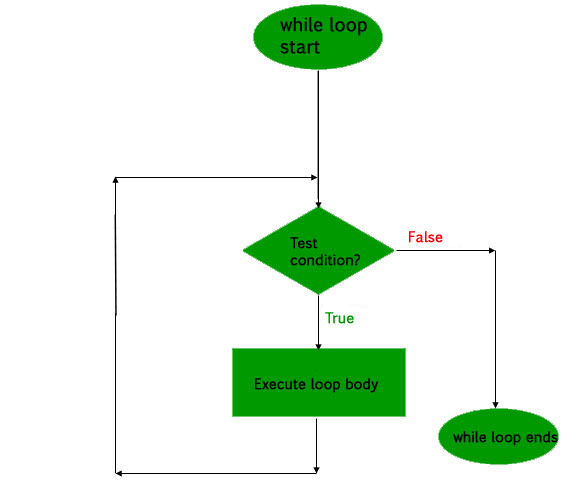# Kotlin while loop

• Difficulty Level : Medium
• Last Updated : 24 Sep, 2021

In programming, loop is used to execute a specific block of code repeatedly until certain condition is met. If you have to print counting from 1 to 100 then you have to write the print statement 100 times. But with help of loop you can save time and you need to write only two lines.

While loop –
It consists of a block of code and a condition. First of all the condition is evaluated and if it is true then execute the code within the block. It repeats until the condition becomes false because every time the condition is checked before entering into the block. The while loop can be thought of as repeating of if statements.

The syntax of while loop-

```while(condition) {
// code to run
}```

Flowchart-Kotlin program to print numbers from 1 to 10 using while loop:
In below program, we print the numbers using while loop. First, initialize the variable number by 1. Put the expression (number <= 10) in while loop and checks is it true or not?. If true, enters in the block and execute the print statement and increment the number by 1. This repeats until the condition becomes false.

## Java

 `fun main(args: Array) {``    ``var number = ``1` `    ``while``(number <= ``10``) {``        ``println(number)``        ``number++;``    ``}``}`

Output:

```1
2
3
4
5
6
7
8
9
10```

Kotlin program to print the elements of an array using while loop:
In the below program we create an array(names) and initialize with different number of strings and also initialize a variable index by 0. The size of an array can be calculated by using arrayName.size. Put the condition (index < names.size) in the while loop.
If index value less than or equal to array size then it enters into the block and print the name stored at the respective index and also increment the index value after each iteration. This repeats until the condition becomes false.

## Java

 `fun main(args: Array) {``    ``var names = arrayOf(``"Praveen"``,``"Gaurav"``,``"Akash"``,``"Sidhant"``,``"Abhi"``,``"Mayank"``)``    ``var index = ``0` `    ``while``(index < names.size) {``        ``println(names[index])``        ``index++``    ``}``}`

Output:

```Praveen
Gaurav
Akash
Sidhant
Abhi
Mayank```

My Personal Notes arrow_drop_up